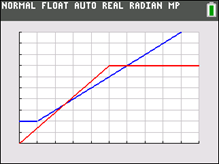# Activities

•• ##### Subject Area

• Math: Precalculus: Systems of Equations

9-12

50 Minutes

• ##### Device
•TI-84 Plus CE

## Which Garage is Better- IB

Updated on 05/25/2022#### Activity Overview

In this activity for the TI-84 family, students are to find the solution to a system of linear equations using technology. They are presented with a problem about parking lot fees.

#### Objectives

• Students will find the solution to a system of linear equations by hand.
• Students will use the TI-84 Plus CE to graph a linear system in two variables and find the intersection point(s).
• Students will create and graph linear piecewise functions.
• Students will try to make a connection with how to understand these topics in IB Mathematics courses and on their final assessments.

#### Vocabulary

• linear system
• piecewise function